# The Problem of the Magnitude of a Magnetic Field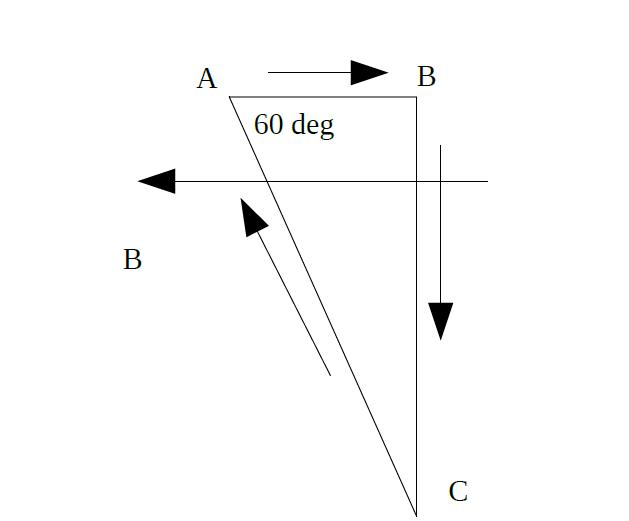A loop wire in the form of the right triangle (see figure) carries a current of 10 A. A magnetic field of 2 T is directly parallel to AB. The length of AB is 3 m. Find the magnitude of the magnetic forces exerted on each side of the loop.

Solution:

1.Magnitude for AB side:

For the AB side, the current is parallel to the magnetic field. Thus, there is no force.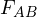= O N

2.Magnitude for BC side:

For the BC side, the current is perpendicular to the magnetic field. The length of BC is:

BC = AB × tan(60°) = 5.2 m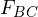= B × I × BC × sin(90°) = 104 N

3.Magnitude for AC side:

For the AC side, the angle between the current of the magnetic field is 60°. The length of the AC side is:

AC = AB / cos(60°) = 6 m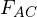= B × I × AC × sin(60°) = 104 N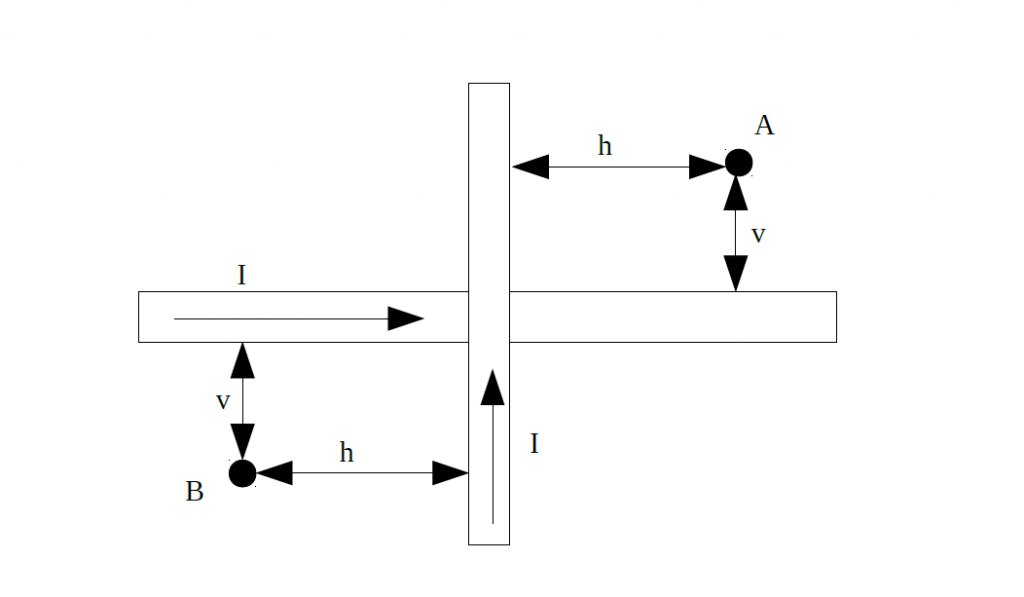Two long and straight wires are perpendicular to each other. The current in each wire is 6 A. Find the magnitudes of magnetic fields and the directions in points A and B, where v is 0.45 m, and h is 0.6 m.

Solution: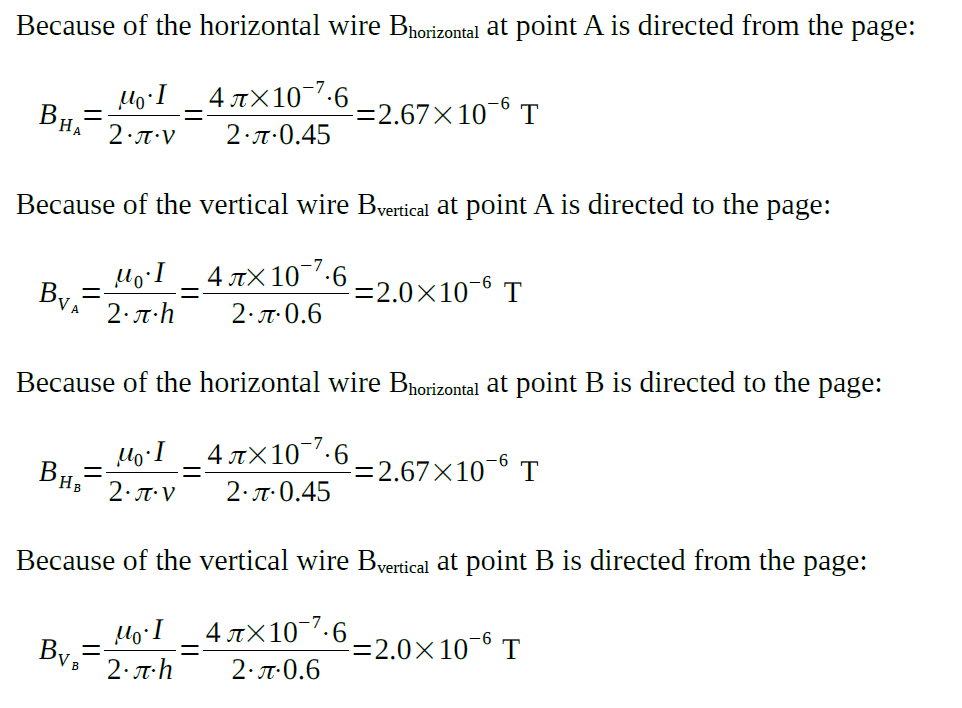Taking the sum of the values from above, we can find the magnitudes of the magnetic fields in points A and B: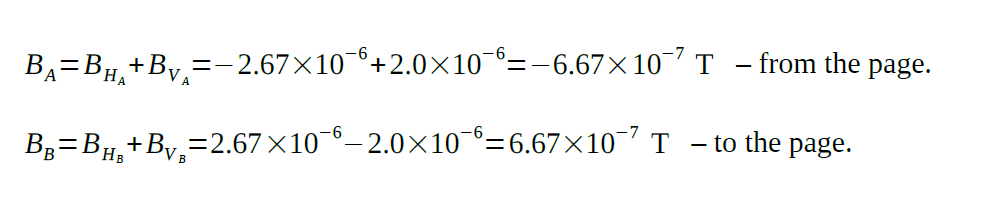Did you find our analysis of the magnitude of magnetic field useful? The experts of AssignmentShark deliver a variety of technical assignments on different technical fields. The scope of our specialization includes engineering, physics, math, chemistry, IT, and many other technical disciplines. Besides the analysis of the magnitude of magnetic force that our specialists generated, there are many more assignments you can find on our website, and even more we can do for you in the future!

The AssignmentShark service provides professional academic assistance for students from all over the world. With over five years working in the industry, we have become synonymous with excellence and reputability and have delivered myriads of successful technical projects to our customers. AssignmentShark is the service you definitely want to try out!